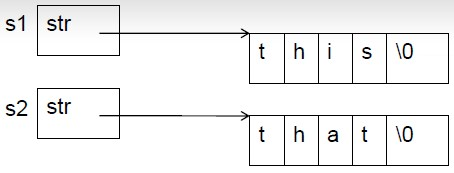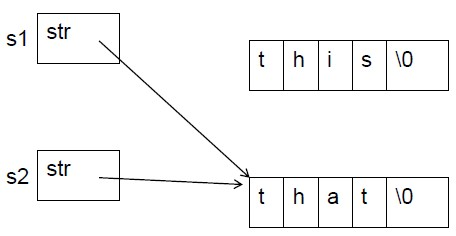2018-09-11  608 views 评论

# C++面向对象程序设计课程笔记（第四周）

## 第一节 运算符重载的基本概念

C++预定义的运算符，只能用于基本数据类型的运算。基本数据类型包括：整型、实型、字符型、逻辑型等。

{

//函数体

}

class Complex{

public:

double real,imag;

Complex(double r = 0.0, double i = 0.0):real(r),imag(i){ }

Complex operator-(const Complex & c);

};

Complex operator+(const Complex &a, const Complex &b){

return Complex(a.real + b.real,a.imag + b.imag);//返回一个临时对象

}

Complex Complex::operator-(const Complex &c)

{

return Complex(real - c.real, imag - c.imag);//返回一个临时对象

}

int main(){

Complex a(4,4),b(1,1),c;

c = a + b;//等价于c = operator+(a,b);

cout << c.real <<"," <<c.imag << endl;

cout<<(a-b).real << "," << (a-b).imag << endl;

//a-b等价于a.operator-(b)

return 0;

}注意：重载为成员函数时，参数个数为运算符目数减一（另一个就是我调用这个成员函数的对象）；重载为普通函数时，参数个数为运算符目数。

## 第二节 赋值运算符的重载

1.赋值运算符“=”的重载

class String{

private:

char * str;//指向动态分配的数组

public:

String():str(new char){str = 0;}

const char * c_str(){return str;};

String & operator = (const char*s);

String::~String(){delete [] str;}

};

String &String::operator = (const char *s)

{

//重载=以使obj="hello"能够成立

delete[] str;

str = new char[strlen(s)+1];

strcpy(str,s);

return * this;

}

int main()

{

String s;

s = "Good luck,";//等价于s.operator=("Good Luck,");

cout<<s.c_str()<<endl;

//String s2 = "hello!";//这条语句不注释掉就会出错

s = "Shenzhou 8!";//等价于s.operator=("Shenzhou 8!");

cout <<s.c_str()<<endl;

return 0;

}

2.相关其它内容

class String{

private:

char * str;//指向动态分配的数组

public:

String():str(new char){str = 0;}

const char * c_str(){return str;};

String & operator = (const char*s){

delete [] str;

str = new char[strlen(s)+1];

strcpy(str,s);

return * this;

};

String::~String(){delete [] str;}

};

String S1,S2;

S1="this";

S2="that";

S1=S2;即S1实际上是指向了S2，两者实际上只指向的一个，原来S1的空间失去了指向，与我们想让S1中的内容（所指向的空间的内容）和S2一样的想法完全不一样。

String s;

s = "Hello";

s = s;

if(this == &s)

return *this;

String(String &s)

{

str = new char[strlen(s.str)+1];

strcpy(str,s.str);

}

## 第三节 运算符重载为友元

class Complex

{

double real,imag;

public:

Complex(double r, double i):real(r),imag(i){};

Complex operator+(double r);

};

Complex Complex::operator+(double r)

{

return Complex(real+r,imag);

}

class Complex

{

double real,imag;

public:

Complex(double r, double i):real(r),imag(i){};

friend Complex operator + (double r, const Complex & c);

};

Complex Complex::operator+(double r)

{

return Complex(real+r,imag);

}

## 第四节 运算符重载实例：可变长整型数组

int main(){//要编写可变长整型数组类，使之能如下使用

CArray a;//开始数组是空的

for(int i = 0; i < 5;++i)

a.push_back(i);//要用动态分配的内存来存放数组元素需要一个指针成员变量

CArray a2,a3

a2 = a;//要重载"="，把a中的值复制给a2

for(int i = 0; i<a.length;++i)

cout<<a2[i]<<"";//要重载[]，因为a2原来是一个对象

a2 = a3;//a2是空的，因为原来的空间被释放了

for(int i = 0;i<a2.length;++i)//a2.length()返回0

cout<<a2[i]<<"";

cout<<endl;

a=100;

CArray a4(a);

CArray A4(A);//要自己写复制构造函数

for(int i = 0; i<a4.length;++i)

cout<<a4[i]<<"";

return 0;

}

class CArray{

int size;//数组元素的个数

int *ptr;//指向动态分配的数组

public:

CArray(int s = 0);//s代表数组元素的个数

CArray(CArray &a);

~CArray();

void push_back(int v);//用于在数组尾部添加一个元素v

CArray & operator=(const CArray &a);

//用于数组对象间的赋值

int length(){return size;}//返回数组元素个数

int & CArray::operator[](int i)

//返回值不能为int，不支持a[i]=4，双目运算符，但是在类内，只有一个运算符

{//用以支持根据下标访问数组元素，如n=a[i]和a[i]=4这样的语句

return ptr[i];

}

};

CArray::CArray(int s):size(s)

{//构造函数

if(s ==0)

ptr = NULL;

else

ptr = new int[s];

}

CArray::CArray(CArray &a){//复制构造函数，要实现深复制

if(!a.ptr){

ptr = NULL;

size = 0;

return;

}

ptr = new int [a.size];

memcpy(ptr,a.ptr,sizeof(int)*a.size);

size = a.size;

}

CArray：：~CArray()

{

if(ptr) delete [] ptr;

}

CArray & CArray::operator=(const CArray &a)//深拷贝，而不是浅拷贝

{//赋值号的作用是使“=”左边对象里存放的数组，大小和内容都和右边的对象一致。

if(ptr == a.ptr)

return *this;//防止前文所述的出错

if(a.ptr == NULL){//如果a里面的数组是空的

if(ptr) delete[] ptr;

ptr = NULL;

size =0;

return *this;

}

if(size <a.size){//如果原有空间不够，则新建一个足够大的空间

//如果足够大，就不分配新的空间直接执行if后面的语句

if(ptr)

delete [] ptr;

ptr = new int[a.size];

}

memcpy(ptr,a.ptr,sizeof(int)*a.size);//空间大小为数目*一个int的字节数

size = a.size;

return *this;

}

void CArray::push_back(int v)

{//在数组尾部添加一个元素。先判断原来是否有元素，如果有元素，就新建一个临时空间，

//然后把原来的元素复制过来，然后删除原来的空间，然后把ptr指针指向了tmpPtr这个临时空间

//这个元素非常浪费资源

if(ptr){

int *tmpPtr = new int[size+1];//重新分配空间

memcpy(tmpPtr,ptr,sizeof(int)*size);//拷贝原数组内容

delete[] ptr;

ptr = tmpPtr;

}

else

ptr = new int;//数组原来是空的

ptr[size++] = v;//加入新的数组元素

}

## 第五节 流插入运算符和流提取运算符的重载

1.流插入运算符的重载

cout是在iostream中定义的ostream的对象。之所以<<能用在cout上是因为，在iostream中对<<进行了重载。

ostream & ostream::operator<<(int n)

{

//代码

return *this;

}

ostream & ostream::operator<<(const char *s)

{

//代码

return *this;

}

cout<<5<<”this”本质上的函数调用形式是：

cout.operator<<(5).operator<<("this");

1：假定下面程序输出为5hello，该补写些什么？

class CStudent{

public: int nAge;

};

int main(){

CStudent s;

s.nAge = 5;

cout << s <<"hello";

return 0;

}

ostream & operator<<(ostream & o, const CStudent & s){

o<<s.nAge;

return o;

}

int main(){

Complex c;

int n;

cin >> c >> n;

cout << c << "," <<n;

return 0;

}

#include <iostream>

#include <string>

#include <cstdlib>

using namespace std;

class Complex{

double real,imag;

public:

Complex(double r=0,double i =0):real(r),imag(i){};

friend ostream & operator<<(ostream & os, const Complex &c);

friend istream & operator >>(istream & is, Compex & c);

};

ostream & operator <<(ostream & os, const Complex & c)

{

os<<c.real<<"+"<<c.imag<<"i";

return os;

}

istream & operator >>(istream & is, Complex & c)

{

string s;

is >> s;//将"a+bi"作为字符串读入，中间不能有空格

int pos = s.find("+",9);

string sTmp = s.substr(0,pos);//分离出代表实部的字符串

c.real = atof(sTmp.c_str());//atof库函数能讲const char*指针指向的内容转换成float

sTmp = s.substr(pos+1,s.length()-pos-2);//分离出代表虚部的字符串

c.imag = atof(sTmp.c_str());

return is;

}

int main(){

Complex c;

int n;

cin >> c >> n;

cout << c << "," <<n;

return 0;

}

C 可以将“<<”重载为全局函数，第一个参数以及返回值，类型都是ostream &

## 第六节 类型转换运算符的重载

#include <iostream>

using namespace std;

class Complex{

double real,imag;

public:

Complex(double r=0,double i =0):real(r),imag(i){};

operator double(){return real;}//类型转换运算符重载时不写返回值类型，因为返回值类型就是它本身

};

int main()

{

Complex c(1.2,3.4);

cout << (double)c <endl;//输出1.2

double n = 2 + c;//c被自动用类型转换运算符，等价与double n = 2+c.operator double()

cout << n;//输出3.2

}

## 第七节 自增自减运算符的重载

（1）前置运算符作为一元运算符重载：

T & operator++()

T & operator—()

T1 & operator++(T2)

T1 & operator—(T2)

（2）后置运算符作为二元运算符重载，多写一个没用的参数int：

T operator++(int)

T operator--(int)

T1 operator++(T2, int)

T1 operator--(T2, int)

int main()

{

CDemo d(5);

cout<(d++) <<",";//等价于d.operator++(0);

cout << d << ",";

cout << (++d) << ",";//等价于d.operator++();

cout << d << endl;

cout << (d--) << ",";//等价于d.operator--(0);

cout << d << ",";

cout << (--d) << ",";//等价于d.operator--();

cout << d << endl;

return 0;

}

5,6,7,7

7,6,5,5

class CDemo{

int n;

public:

CDemo(int i=0):n(i){}

CDemo & operator++();//前置形式++n返回值就是n的引用，所以这里要用引用

CDemo operator++(int);//后置形式，n++返回的是一个临时变量，所以这里不能用引用

operator int(){return n;}

friend CDemo & operator--(CDemo &);

friend CDemo operator--(CDemo &, int);

};

CDemo & CDemo::operator++():

{//前置

n ++;

return *this;

}

CDemo CDemo::operator++(int k):

{//后置

CDemo tmp(*this);//记录修改前的对象

n++;

return tmp;//返回修改前的对象

}//s++即为s.operator++(0);

CDemo & operator--(CDemo & d){//前置

d.n--;

return d;

}

CDemo operator--(CDemo &d, int){//后置

CDemo tmp(d);

d.n--;

return tmp;

}//s--即为operator--(s,0)

1.C++不允许定义新的运算符；

2.重载后运算符的含义应该符合日常习惯；

3.运算符重载不改变运算符的优先级；

4.以下运算符不能被重载：“.”、“.*”、“::”、“?:”、sizeof；

5.重载运算符()、[]、->或者赋值运算符=时，运算符重载函数必须生命为成员函数。